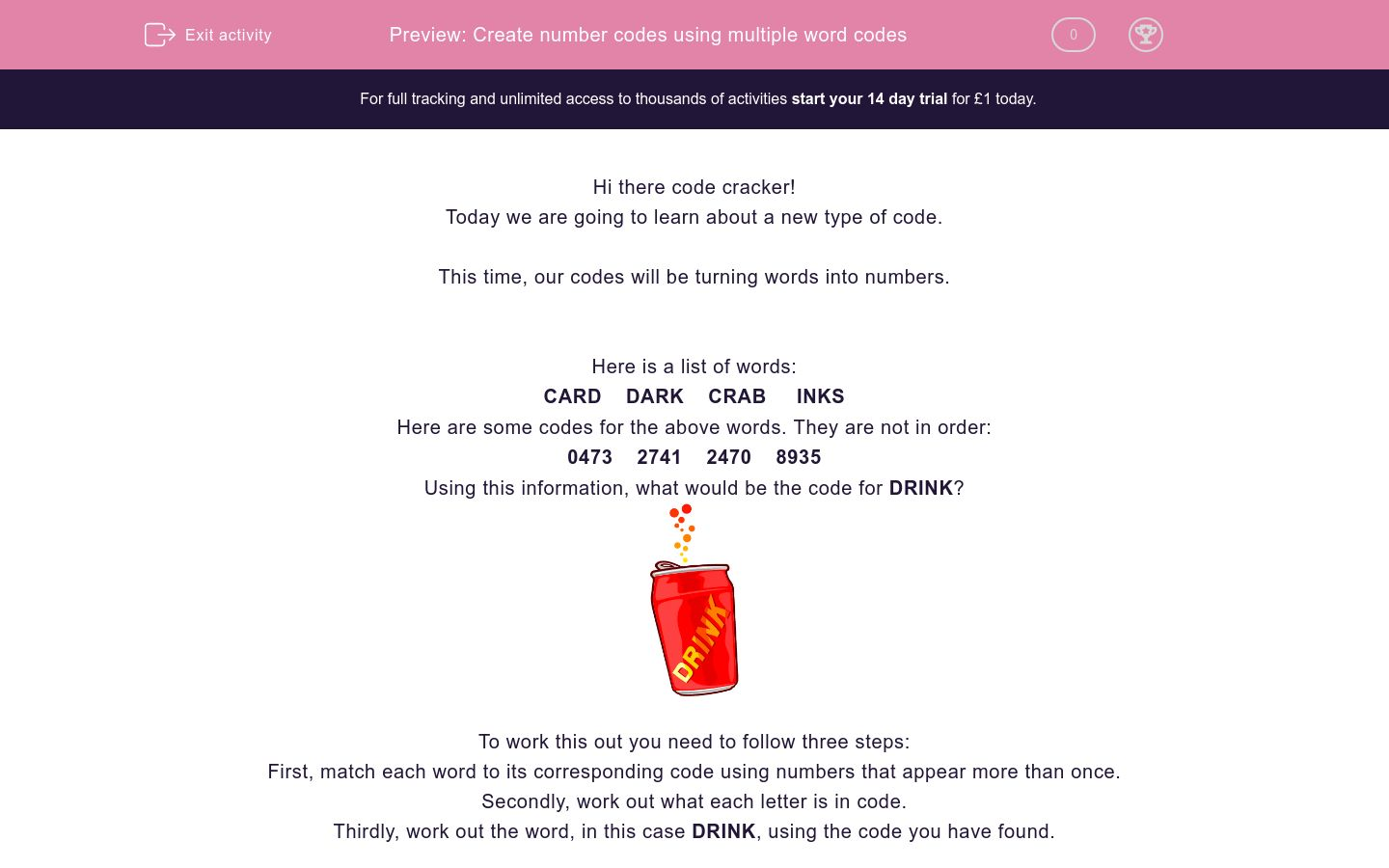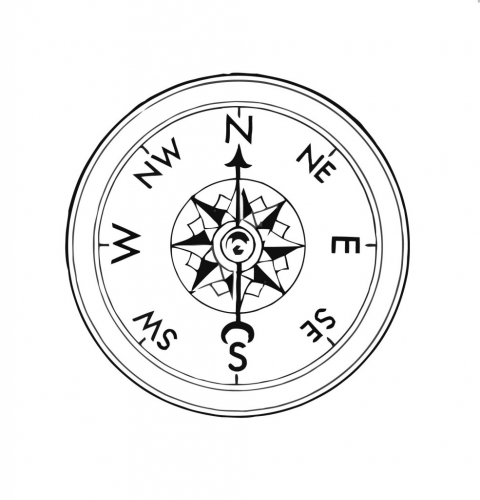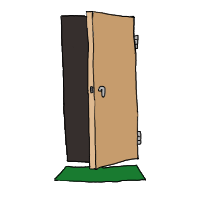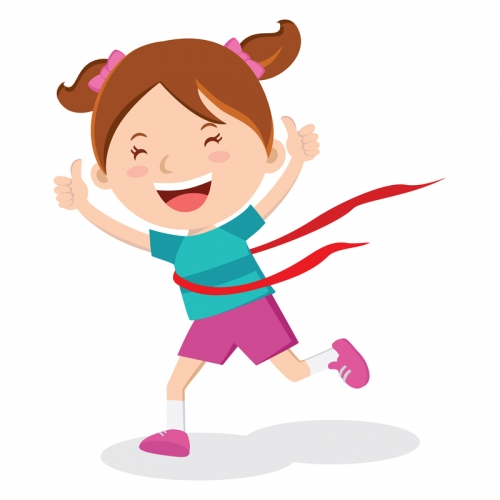# Create number codes using multiple word codes

In this worksheet, students will turn words into number codes using multiple words and codes to find and apply the pattern. It will develop their encoding and problem solving skills.Key stage:  KS 2

Curriculum topic:   Verbal Reasoning

Curriculum subtopic:   Number Code Breaking

Difficulty level:### QUESTION 1 of 10

Hi there code cracker!

Today we are going to learn about a new type of code.

This time, our codes will be turning words into numbers.

Here is a list of words:

CARD    DARK    CRAB     INKS

Here are some codes for the above words. They are not in order:

0473    2741    2470    8935

Using this information, what would be the code for DRINK?To work this out you need to follow three steps:

First, match each word to its corresponding code using numbers that appear more than once.

Secondly, work out what each letter is in code.

Thirdly, work out the word, in this case DRINK, using the code you have found.

So let’s give it a try!

There are two codes beginning with the number 2. Do we have any words starting with the same letter?

We have two words starting with the letter C, so 2 must represent C (2=C).

We have two codes with the number 4 in the second letter position. Which letter appears in the second letter position twice? 4 must be the letter A (4=A).

We now have enough information to match each word to a code, giving us:

DARK = 0473

CRAB = 2741

CARD = 2470

INKS = 8935

So D = 0, R = 7, I = 8, N = 9, K = 3.

If we use this code on the word DRINK, we get the answer of 07893.

In this activity, you will be a code cracker and work out the digit-based code for a given word.

It can be really helpful to write the words out and then write the corresponding numbers above each letter as you work them out.  Give it a go!

Let’s get started!

Welcome back to number code cracking, code cracker! You are now at Year 5 Level - amazing work! In this worksheet, we are going to focus on mastering the number code cracking skill needed in Year 5. The next two levels will focus on this and on building your vocabulary.

So, let's...Look at the list of words below:

PEA  APE  AIM

Here are some codes for the above words. They are not necessarily in order:

263  632  241

Using this information, what would be the code for MAP?

OK, let's try practising that skill again.Look at the list of words below:

FOR  FOG  LOG

Here are some codes for the above words. They are not necessarily in order:

239  230  630

Using this information, what would be the code for ROLL?

Brilliant work so far! Let's have another crack at doing some code cracking!Look at the list of words below:

TOW  SEW  TOE

Here are some codes for the above words. They are not necessarily in order:

532  192  193

Using this information, what would be the code for WEST?

Brilliant work so far! Remember, this skill can take a while to get right, so don't worry if you are still getting some questions wrong. I know you are going to be a number code cracking superstar in the end!Look at the list of words below:

FOG  ROD  ARE

Here are some codes for the above words. They are not necessarily in order:

038  739  604

Using this information, what would be the code for GRADE?

OK, code cracker, we are officially halfway through the worksheet! Great work so far - let's keep working through it together. You've got this!Look at the list of words below:

YELL  SLOW  PLAY

Here are some codes for the above words. They are not necessarily in order:

2941  7953  3899

Using this information, what would be the code for SLAY?

You are really getting there with this new skill, code cracker, well done! Now, time to show me what you're made of  - on question 6!Look at the list of words below:

DRY  FOR  FRY

Here are some codes for the above words. They are not necessarily in order:

529  362  329

Using this information, what would be the code for DOOR?

Are you feeling more confident, code cracker? Remember, whenever you need help you can just ask to see the answer below and I'll be there to explain it to you. You're not alone!Look at the list of words below:

BOW  LOW  WEB

Here are some codes for the above words. They are not necessarily in order:

139  851  951

Using this information, what would be the code for BOWL?

I can't believe we only have three questions left, code cracker. Amazing work - it's almost time to celebrate!Look at the list of words below:

CRY  TRY  CUT

Here are some codes for the above words. They are not necessarily in order:

352  752  713

Using this information, what would be the code for YURT?

This is the penultimate question, code cracker - look how far you've come! Well done!Look at the list of words below:

SEEM  MEET  TEAM

Here are some codes for the above words. They are not necessarily in order:

6229  9221  1259

Using this information, what would be the code for SEAT?

You made it, code cracker! Amazing work! You should feel so proud of yourself for making it all the way to the end of the sheet.Let's get this last question done!

Look at the list of words below:

ABLE  BLOW  BANE

Here are some codes for the above words. They are not necessarily in order:

9240  9651  6921

Using this information, what would be the code for OWN?

• Question 1

Welcome back to number code cracking, code cracker! You are now at Year 5 Level - amazing work! In this worksheet, we are going to focus on mastering the number code cracking skill needed in Year 5. The next two levels will focus on this and on building your vocabulary.

So, let's...Look at the list of words below:

PEA  APE  AIM

Here are some codes for the above words. They are not necessarily in order:

263  632  241

Using this information, what would be the code for MAP?

126
EDDIE SAYS
How are you getting on, code cracker? I hope this is becoming a bit more familiar. Do check through the explanation carefully! 1. Match each word to its corresponding code using numbers that appear more than once: There are two codes with the number 2 in the first letter position. We have two words with A as the first letter. Therefore, 2 must be A (2 = A). This is all we need to work out the codes, code cracker! The number 2 also appears in the code 632, so we know this must be the code for the word PEA. We can work out the other two codes from this! We now have enough information to match each word to a code, giving us: PEA = 632 APE = 263 AIM = 241 2. Work out what each letter is in code: So M = 1, A = 2, P = 6 3. Work out the word: If we use this code on the word MAP, we get the answer of 126.
• Question 2

OK, let's try practising that skill again.Look at the list of words below:

FOR  FOG  LOG

Here are some codes for the above words. They are not necessarily in order:

239  230  630

Using this information, what would be the code for ROLL?

9366
EDDIE SAYS
I hope that all this practice is helping! Remember: if you get a question wrong, have another go at it using the explanation to help you! 1. Match each word to its corresponding code using numbers that appear more than once: There are two codes with the number 2 in the first letter position. We have two words with F as the first letter. Therefore, 2 must be F (2 = F). The number 3 appears in the second letter position in all three words. The letter O is the second letter in all three words. Therefore, 3 is O (3 = O). The code 630 does NOT contain the number 2 (remember: 2 = F), so that must be the code for LOG. Does that make sense, code cracker? We now have enough information to match each word to a code, giving us: FOR = 239 FOG = 230 LOG = 630 2. Work out what each letter is in code: So R = 9, O = 3, L = 6, L= 6 3. Work out the word: If we use this code on the word ROLL, we get the answer of 9366.
• Question 3

Brilliant work so far! Let's have another crack at doing some code cracking!Look at the list of words below:

TOW  SEW  TOE

Here are some codes for the above words. They are not necessarily in order:

532  192  193

Using this information, what would be the code for WEST?

2351
EDDIE SAYS
Did you move in the right direction with this one, code cracker? I hope you are feeling more confident! Remember to read the explanations carefully - I'm here to help! 1. Match each word to its corresponding code using numbers that appear more than once: The number 2 is the final number in two codes and the letter W is the final letter in two of the words. Therefore, 2 must be W (2 = W). The number 1 appears in the first letter position in two words. The letter T is the first letter of two of the words. Therefore, 1 is T (1 = T). So, now we can move on to working out these codes, code cracker: SEW = 532 TOW = 192 TOE = 193 2. Work out what each letter is in code: So W = 2, E = 3, S = 5, T = 1. 3. Work out the word: If we use this code on the word WEST, we get the answer of 2351.
• Question 4

Brilliant work so far! Remember, this skill can take a while to get right, so don't worry if you are still getting some questions wrong. I know you are going to be a number code cracking superstar in the end!Look at the list of words below:

FOG  ROD  ARE

Here are some codes for the above words. They are not necessarily in order:

038  739  604

Using this information, what would be the code for GRADE?

90684
EDDIE SAYS
Did you make the GRADE? I think you are doing brilliantly, code cracker! Let's go through this carefully: 1. Match each word to its corresponding code using numbers that appear more than once: The number 3 is in the second letter position in two codes and the letter O is in the second letter position in two of the words. Therefore, 3 must be O (3 = O). The number 0 is in the first letter position in one of the words and the second letter position in another. The letter R is the first letter of ROD and the second letter of ARE. Therefore, 0 must be R (0 = R). So, now we can move on to working out these codes, code cracker: FOG = 739 ROD = 038 ARE = 604 2. Work out what each letter is in code: So G = 9, R = 0, A = 6, D = 8, E = 4. 3. Work out the word: If we use this code on the word GRADE, we get the answer of 90684.
• Question 5

OK, code cracker, we are officially halfway through the worksheet! Great work so far - let's keep working through it together. You've got this!Look at the list of words below:

YELL  SLOW  PLAY

Here are some codes for the above words. They are not necessarily in order:

2941  7953  3899

Using this information, what would be the code for SLAY?

2953
EDDIE SAYS
You are SLAYing this worksheet, code cracker! Fantastic work! Keep taking the time to read the explanations to check your work! 1. Match each word to its corresponding code using numbers that appear more than once: The number 9 appears in the second letter position in two codes. The letter L appears as the second letter in two of the words. So, 9 must be L (9 = L). We now have everything we need to work out the rest, code cracker! Because we know that 9 = L, we know that the code 3899 must be the code for YELL (it is the only word with a double L at the end of it). So, now we can move on to working out these other codes, code cracker: YELL = 3899 SLOW = 2941 PLAY = 7953 2. Work out what each letter is in code: So S = 2, L = 9, A = 5, Y = 3. 3. Work out the word: If we use this code on the word SLAY, we get the answer of 2953.
• Question 6

You are really getting there with this new skill, code cracker, well done! Now, time to show me what you're made of  - on question 6!Look at the list of words below:

DRY  FOR  FRY

Here are some codes for the above words. They are not necessarily in order:

529  362  329

Using this information, what would be the code for DOOR?

5662
EDDIE SAYS
OK, code cracker, let's see if we can get to the bottom of this number code cracking challenge! We'll follow our usual technique: 1. Match each word to its corresponding code using numbers that appear more than once: The number 9 is in the third letter position in two of the codes. The letter Y is in the third letter position in two of the words. Therefore, 9 must be Y (9 = Y). The number 3 appears in the first letter position in two words. The letter F is the first letter of two of the words. Therefore, 3 is F (3 = F). That's all that we need! So, now we can move on to working out these codes, code cracker: DRY = 529 FOR = 362 FRY = 329 Now then, onto the next stage! 2. Work out what each letter is in code: So D = 5, O = 6, O = 6, R = 2. 3. Work out the word: If we use this code on the word DOOR, we get the answer of 5662.
• Question 7

Are you feeling more confident, code cracker? Remember, whenever you need help you can just ask to see the answer below and I'll be there to explain it to you. You're not alone!Look at the list of words below:

BOW  LOW  WEB

Here are some codes for the above words. They are not necessarily in order:

139  851  951

Using this information, what would be the code for BOWL?

9518
EDDIE SAYS
OK, code cracker, let's see if we can get to grips with this conundrum! I know you can do it! 1. Match each word to its corresponding code using numbers that appear more than once: The number 1 appears in the third letter position in two codes and the letter W is the third letter in two of the words. Therefore, 1 must be W (1 = W). That's all we need, code cracker! We see that there is a code beginning with 1, which is 139. If 1 = W, then that must be the code for WEB and we can work out the codes for the other words from there! So, now we can move on to working out these codes, code cracker: BOW = 951 LOW = 851 WEB = 139 2. Work out what each letter is in code: So B = 9, O = 5, W = 1, L = 8. 3. Work out the word: If we use this code on the word BOWL, we get the answer of 9518. Nice work, code cracker! Onto the next!
• Question 8

I can't believe we only have three questions left, code cracker. Amazing work - it's almost time to celebrate!Look at the list of words below:

CRY  TRY  CUT

Here are some codes for the above words. They are not necessarily in order:

352  752  713

Using this information, what would be the code for YURT?

2153
EDDIE SAYS
OK, let's follow our usual technique, code cracker! Hopefully, you'll find working through each of the stages simplifies what can sometimes be a challenging skill to master. 1. Match each word to its corresponding code using numbers that appear more than once: The number 7 appears in the first letter position in two codes and the letter C is in the first letter position in two words. Therefore, 7 must be C (7 = C). The number 5 appears in the second letter position in two of the codes. The letter R is the second letter of two of the words. Therefore, 5 is R (5 = R). From this, we can work out the rest of the codes! So, now we can move on to working out these codes, code cracker: CRY = 752 TRY = 352 CUT = 713 2. Work out what each letter is in code: So Y = 2, U = 1, R = 5, T = 3. 3. Work out the word: If we use this code on the word YURT, we get the answer of 2153. Have you come across the word yurt before? It is the name for a large circular tent, often made from animal skins, and used in countries like Mongolia and Siberia.
• Question 9

This is the penultimate question, code cracker - look how far you've come! Well done!Look at the list of words below:

SEEM  MEET  TEAM

Here are some codes for the above words. They are not necessarily in order:

6229  9221  1259

Using this information, what would be the code for SEAT?

6251
EDDIE SAYS
Almost there, code cracker, fantastic! We do need to make sure we go through this carefully, though, and not rush to get to the end! We'll use our usual technique: 1. Match each word to its corresponding code using numbers that appear more than once: The number 9 appears in the fourth letter position in two codes and the letter M is in the fourth letter position in two words. Therefore, 9 must be M (9 = M). The number 2 appears in the second and third letter position in two of the codes. The letter E is the second letter of three of the words and the third letter of two of the words. Therefore, 2 is E (2 = E). From this, we can work out the rest of the codes! So, now we can move on to working out these codes, code cracker: SEEM = 6229 MEET = 9221 TEAM = 1259 2. Work out what each letter is in code: So S = 6, E = 2, A = 5, T = 1. 3. Work out the word: If we use this code on the word SEAT, we get the answer of 6251.
• Question 10

You made it, code cracker! Amazing work! You should feel so proud of yourself for making it all the way to the end of the sheet.Let's get this last question done!

Look at the list of words below:

ABLE  BLOW  BANE

Here are some codes for the above words. They are not necessarily in order:

9240  9651  6921

Using this information, what would be the code for OWN?

405
EDDIE SAYS
You have done so well, code cracker! Make sure you take some time to feel very proud of your progress! Now, let's work carefully through our last explanation: 1. Match each word to its corresponding code using numbers that appear more than once: The number 9 appears in the first letter position in two codes and the letter B is in the first letter position in two words. Therefore, 9 must be B (9 = B). The number 1 appears in the fourth letter position in two of the codes. The letter E is the fourth letter of two of the words. Therefore, 1 is E (1 = E). From this, we can work out the rest of the codes! So, now we can move on to working out these codes, code cracker: ABLE = 6921 BLOW = 9240 BANE = 9651 2. Work out what each letter is in code: So O = 4, W = 0, N = 5 3. Work out the word: If we use this code on the word OWN, we get the answer of 405.
---- OR ----

Sign up for a £1 trial so you can track and measure your child's progress on this activity.

### What is EdPlace?

We're your National Curriculum aligned online education content provider helping each child succeed in English, maths and science from year 1 to GCSE. With an EdPlace account you’ll be able to track and measure progress, helping each child achieve their best. We build confidence and attainment by personalising each child’s learning at a level that suits them.

Get started